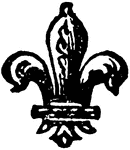### The Fleur-de-lis

The heraldic charge borne by the sixth eldest son in a family.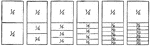### Fraction Comparisons

Rectangles comparing fraction values to one half: 1/4, 1/6, 1/8, 1/10, and 1/12.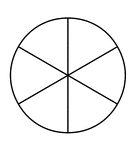### Fraction Pie Divided into Sixths

A circle divided into sixths.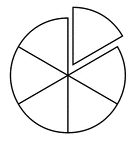### Fraction Pie Divided into Sixths

A circle divided into sixths with one sixth separated.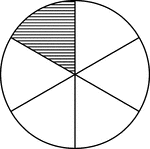### Fraction Pie Divided into Sixths

A circle divided into sixths with one sixth shaded.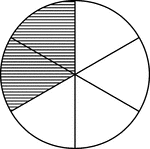### Fraction Pie Divided into Sixths

A circle divided into sixths with two sixths shaded.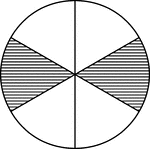### Fraction Pie Divided into Sixths

A circle divided into sixths with two sixths shaded.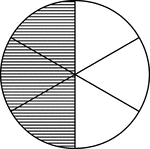### Fraction Pie Divided into Sixths

A circle divided into sixths with three sixths shaded.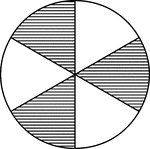### Fraction Pie Divided into Sixths

A circle divided into sixths with three sixths shaded.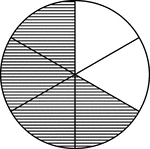### Fraction Pie Divided into Sixths

A circle divided into sixths with four sixths shaded.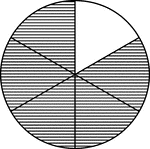### Fraction Pie Divided into Sixths

A circle divided into sixths with five sixths shaded.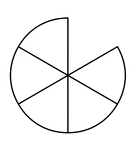### Five Sixths of a Fraction Pie

Five sixths of a circle.### Four Sixths of a Fraction Pie

Four sixths of a circle.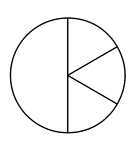### One Half and Three Sixths of a Pie Fraction

A circle subdivided into one half and three sixths.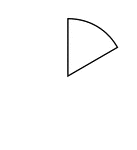### One Sixth of a Fraction Pie

One sixth of a circle.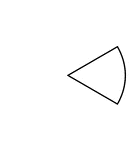### One Sixth of a Fraction Pie

One sixth of a circle.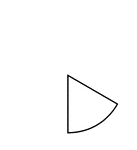### One Sixth of a Fraction Pie

One sixth of a circle.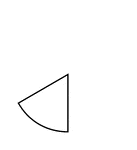### One Sixth of a Fraction Pie

One sixth of a circle.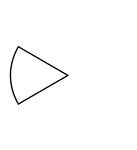### One Sixth of a Fraction Pie

One sixth of a circle.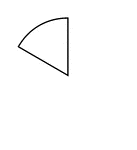### One Sixth of a Fraction Pie

One sixth of a circle.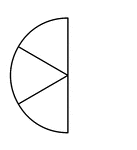### Three Sixths of a Fraction Pie

Three sixths of a circle.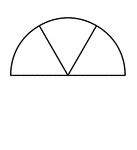### Three Sixths of a Fraction Pie

Three sixths of a circle.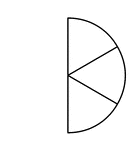### Three Sixths of a Fraction Pie

Three sixths of a circle.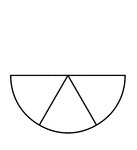### Three Sixths of a Fraction Pie

Three sixths of a circle.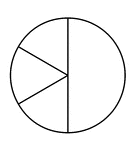### Three Sixths and One Half of a Pie Fraction

A circle subdivided into three sixths and one half.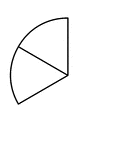### Two Sixths of a Fraction Pie

Two sixths of a circle.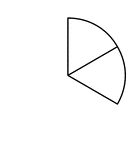### Two Sixths of a Fraction Pie

Two sixths of a circle.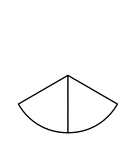### Two Sixths of a Fraction Pie

Two sixths of a circle.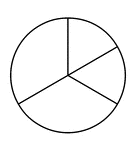### Two Thirds and Two Sixths of a Pie Fraction

A circle subdivided into two thirds and two sixths.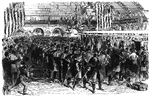### The Sixth regiment

Thousands of patriotic citizens filled every available space in the big railroad station in Jersey City…